# How to denote matrix in LaTeX?

Matrix is ​​an array and table where numbers, symbols, and expressions are arranged along the row and column according to the sequence. And these numbers, symbols, and expressions are called elements of the matrix.

Before defining a matrix in latex, you need to understand the structure of the matrix well.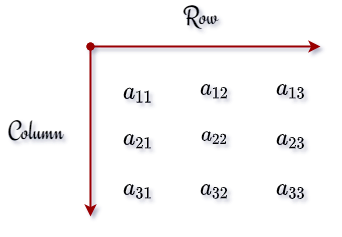## Define matrix in LaTeX

1 First, to define a matrix in latex, you need to create a matrix environment.matrix is ​​passed as an argument between \begin and \end commands. And this argument indicates that the matrix will be bound by which bracket? So, there is more than one argument to define more than one bracket.

2 Second, you need to create rows and columns according to your needs.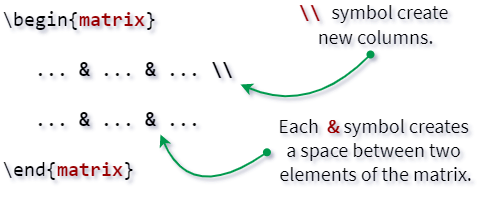& and \\ symbols are used to arrange elements along rows and columns sequentially in a matrix environment. This & symbol arranges the elements of the matrix individually along the row. And \\ symbol creates a new column.

3 Third, the elements of the matrix must be inserted sequentially into the matrix environment. Which will be separated by the & symbol along the row.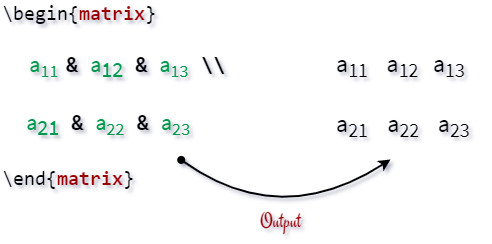And in latex, you can define a complete matrix with the help of the above three steps.

For example, look at the following square matrix.

\documentclass{article}
\usepackage{amsmath}
\begin{document}
$$\begin{matrix} a_{11} & a_{12}\\ a_{21} & a_{22} \end{matrix}$$
\end{document}

Output :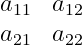The matrix is ​​not specified by default in latex. For this, you need to install the external package amsmath.

## Matrix with different type of brackets in LaTeX

The arranged elements of the matrix are bound by different brackets and no brackets are given on either side of some matrix.

And the arranged elements of the matrix will be bound by brackets depending on the argument. So, take a look at this table below

 pmatrix ( ) bmatrix [ ] Bmatrix { } vmatrix | | Vmatrix || ||

### 1. Matrix with no brackets

If the matrix is ​​not bound by a bracket. In that case, the matrix must be passed as an argument within the matrix environment.

\documentclass{article}
\usepackage{amsmath}
\begin{document}
$$\begin{matrix} a_{11} & a_{12} & a_{13}\\ a_{21} & a_{22} & a_{23}\\ a_{31} & a_{32} & a_{33} \end{matrix}$$
\end{document}

Output :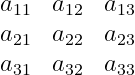### 2. Matrix with parenthesis

If the matrix is ​​surrounded by parenthesis. Then you need to use pmatrix as an argument.

\documentclass{article}
\usepackage{amsmath}
\begin{document}
\begin{equation}
\begin{pmatrix}
a_{11} & a_{12} & a_{13}\\
a_{21} & a_{22} & a_{23}\\
a_{31} & a_{32} & a_{33}
\end{pmatrix}
\end{equation}
\end{document}

Output :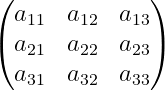### 3. Matrix with square bracket

If the matrix is ​​surrounded by a square bracket. Then you need to pass bmatrix between begin and end command as an argument.

\documentclass{article}
\usepackage{amsmath}
\begin{document}
\begin{equation}
\begin{bmatrix}
a_{11} & a_{12} & a_{13}\\
a_{21} & a_{22} & a_{23}\\
a_{31} & a_{32} & a_{33}
\end{bmatrix}
\end{equation}
\end{document}

Output :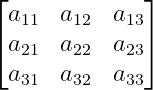### 4. Matrix with curly brackets

Bmatrix should also be used in the case of curly brackets. Latex is a case-sensitive language. In latex, capital letters and small letters are not the same things. Bmatrix and bmatrix are two different arguments for this in latex.

\documentclass{article}
\usepackage{amsmath}
\begin{document}
\begin{equation}
\begin{Bmatrix}
a_{11} & a_{12} & a_{13}\\
a_{21} & a_{22} & a_{23}\\
a_{31} & a_{32} & a_{33}
\end{Bmatrix}
\end{equation}
\end{document}

Output :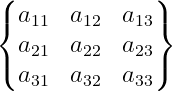### 5. Matrix with vertical bars

You all know when the value of a matrix is ​​determined. In that case, both sides of the matrix are bounded by vertical bars. For this vertical bar, you need to pass the vmatrix argument.

\documentclass{article}
\usepackage{amsmath}
\begin{document}
\begin{equation}
\begin{vmatrix}
a_{11} & a_{12} & a_{13}\\
a_{21} & a_{22} & a_{23}\\
a_{31} & a_{32} & a_{33}
\end{vmatrix}
\end{equation}
\end{document}

Output :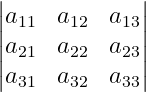### 6. Matrix with double vertical bars

You have noticed that double vertical bars are used on both sides of the matrix. And use Vmatrix as an argument where V will be capital.

\documentclass{article}
\usepackage{amsmath}
\begin{document}
\begin{equation}
\begin{Vmatrix}
a_{11} & a_{12} & a_{13}\\
a_{21} & a_{22} & a_{23}\\
a_{31} & a_{32} & a_{33}
\end{Vmatrix}
\end{equation}
\end{document}

Output :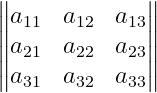If you look at the above programs, you can easily understand which argument is used for which bracket!

## Define different type of matrix in LaTex

The matrix is ​​divided into different types according to the position of the elements in the matrix.

### 1. Square matrix

In this case, you need to take an equal number of rows and columns. Because the number of rows and columns of the square matrix is ​​equal.

\documentclass{article}
\usepackage{amsmath}
\begin{document}
\begin{equation}
\begin{bmatrix}
a_{1,1} & a_{1,2} & \cdots & a_{1,m} \\
a_{2,1} & a_{2,2} & \cdots & a_{2,m} \\
\vdots  & \vdots  & \ddots & \vdots  \\
a_{m,1} & a_{m,2} & \cdots & a_{m,m}
\end{pmatrix}
\end{equation}
\end{document}

Output :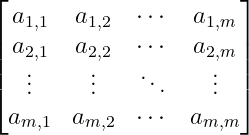### 2. Null matrix

In the case of zero matrix, all the elements of the matrix will be zero.

\documentclass{article}
\usepackage{amsmath}
\begin{document}
\begin{equation}
\begin{bmatrix}
0 & 0 & 0\\
0 & 0 & 0\\
0 & 0 & 0
\end{bmatrix}
\end{equation}
\end{document}

Output :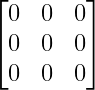### 3. Diagonal matrix

The elements of the diagonal matrix are located along the diagonal.

\documentclass{article}
\usepackage{amsmath}
\begin{document}
\begin{equation}
\begin{bmatrix}
a_{1} &  & \\
& \ddots & \\
&  &  a_{n}
\end{bmatrix}
\end{equation}
\end{document}

Output :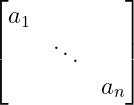### 4. Row matrix

In the case of a row matrix, the elements of the matrix are arranged along a row.

\documentclass{article}
\usepackage{amsmath}
\begin{document}
\begin{equation}
\begin{pmatrix}
a_{1} & \cdots & a_{n}\\
\end{pmatrix}
\end{equation}
\end{document}

Output :### 5. Column matrix

In the same way, the elements of the column matrix are located along a column.

\documentclass{article}
\usepackage{amsmath}
\begin{document}
\begin{equation}
\begin{bmatrix}
a_{1} \\ \vdots \\ a_{n}
\end{bmatrix}
\end{equation}
\end{document}

Output :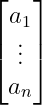### 6. Vertical matrix

In the case of vertical matrix, the number of rows will be more than the number of columns.

\documentclass{article}
\usepackage{amsmath}
\begin{document}
\begin{equation}
\begin{bmatrix}
a_{11} & a_{12}\\
a_{21} & a_{22}\\
a_{31} & a_{32}\\
a_{41} & a_{42}
\end{bmatrix}
\end{equation}
\end{document}

Output :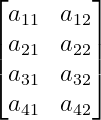### 7. Horizontal matrix

In the horizontal matrix, the number of rows will be less than the number of columns.

\documentclass{article}
\usepackage{amsmath}
\begin{document}
\begin{equation}
\begin{bmatrix}
a_{11} & a_{12}\\
a_{21} & a_{22}\\
a_{31} & a_{32}\\
a_{41} & a_{42}
\end{bmatrix}
\end{equation}
\end{document}

Output :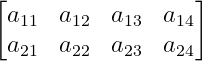There are many types of matrix except the above matrix. Hopefully, with the help of latex, you will be able to represent other types of matrix in the same way.

## Use physics package for matrix

You can represent the matrix using the physics package instead of the amsmath package. Where the \matrixquantity command is used to denote the matrix. This \matrixquantity command is written as \mqty in short form.

And pass the body of the matrix as an argument in \mqty command which consists of a combination of row and column. Notice this example below

\documentclass{article}
\usepackage{physics}
\begin{document}
$$\mqty{a & b \\ c & d}$$
\end{document}

Output :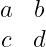You can use different types of brackets on both sides of the matrix. However, the syntax of the command will be different for different brackets.

\documentclass{article}
\usepackage{physics}
\begin{document}
$$\mqty{a & b \\ c & d} \quad \mqty(a & b \\ c & d) \quad \mqty*(a & b \\ c & d) \quad \mqty[a & b \\ c & d] \quad \mqty|a & b \\ c & d|$$
\end{document}

Output :### 2 thoughts on “How to denote matrix in LaTeX?”

1.agar hm matrix main matrix k sath numbering dena chahty ho column num or rows ko matrix pa e to wo kaise latix main add ho ga?
kindly plx bta dye.

1.In the image below, did you want this kind of output? Where the equation number will come with the matrix! Please confirm this ???